# Exercise 15.2 Introduction to Graphs- NCERT Solutions Class 8

## Chapter 15 Ex.15.2 Question 1

Plot the following points on a graph sheet. Verify if they lie on a line

(a)

$$\rm{A(4,0),{{B}}(4,2),{{C}}(4,6),{{D}}(4,2.5)}$$

(b)

$$\rm{P(1,1),\;{{Q}}(2,2),\;{{R}}(3,3),\;{{S}}(4,4)}$$

(c)

$$\rm{K(2,3),\; L( (5,3),\;M(5,5),\;N(2,5)}$$

### Solution

Reasoning:

Consider $$x$$-axis and $$y$$-axis as a number line starting from ($$0,0$$). And locate the given points.

Steps:

(a) We can plot the given points and join the consecutive points on a graph paper as follows.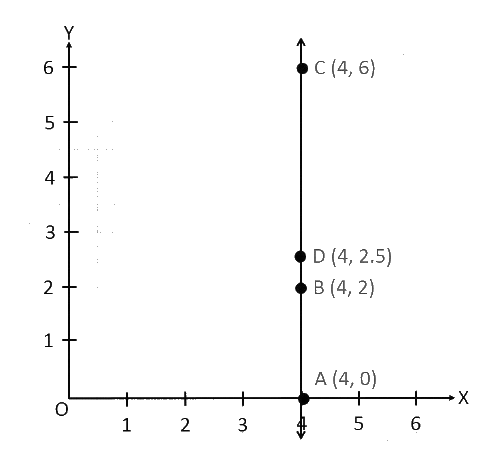From the graph, it can be observed that the points $$A, B, C,$$ and $$D$$ lie on the same line.

(b) We can plot the given points and join the consecutive points on a graph paper as follows.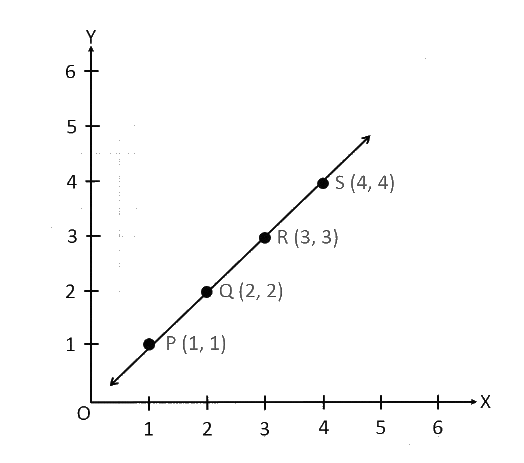Hence, points $$P, Q, R,$$ and $$S$$ lie on the same line.

(c) We can plot the given points and join the consecutive points on a graph paper as follows.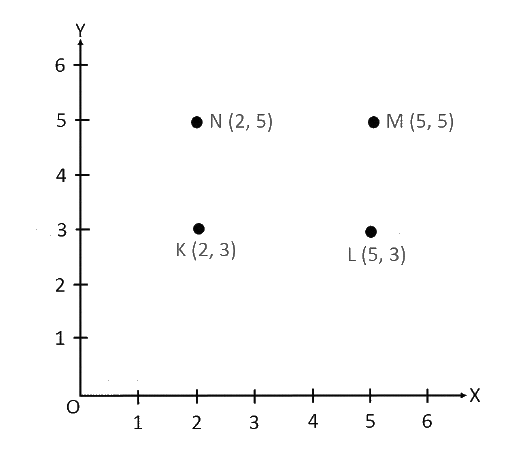Hence, points$$K, L, M,$$and $$N$$ are not lying on the same line.

## Chapter 15 Ex.15.2 Question 2

Draw the line passing through ($$2, 3$$) and ($$3, 2$$). Find the coordinates of the points at which this line meets the $$x$$-axis and $$y$$-axis.

### Solution

Steps: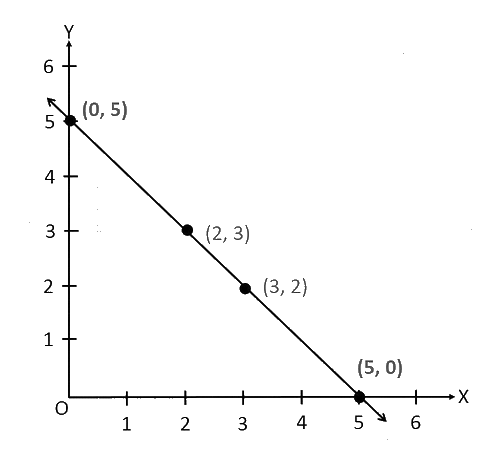From the graph, it can be observed that the line joining the points ($$2, 3$$) and ($$3, 2$$) meets the x-axis at the point ($$5, 0$$) and the y-axis at the point ($$0, 5$$).

## Chapter 15 Ex.15.2 Question 3

Write the coordinates of the vertices of each of these adjoining figures.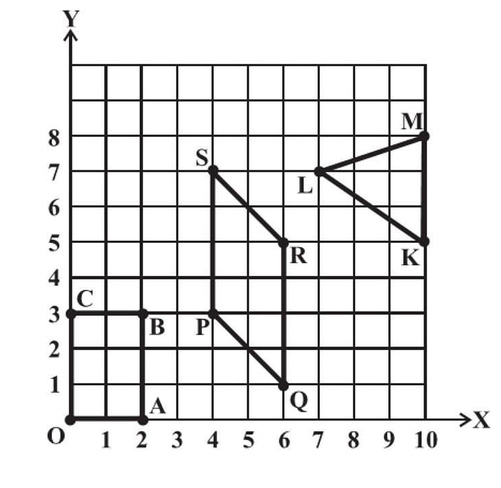### Solution

Reasoning:

$$x$$-axis and $$y$$-axis are represented as a number line starting from ($$0,0$$). Locate the numerical value of the given points.

Steps:

The coordinates of the vertices in the given figure are as follows.

\begin{align}&{{O(0,0), A(2,0), B(2,3),C(0,3)}}\\&{{P(4,3), Q(6,1), R(6,5),S(4,7)}}\\&{{K(10,5), L(7,7),M (10,8)}}\end{align}

## Chapter 15 Ex.15.2 Question 4

State whether True or False. Correct those are false.

(i) A point whose $$x$$ coordinate is zero and $$y-$$coordinate is non-zero will lie on the $$y-$$axis.

(ii) A point whose $$y$$ co-ordinate is zero and $$x$$-coordinate is $$5$$ will lie on $$y$$-axis.

(iii) The coordinates of the origin are ($$0, 0$$).

### Solution

Steps:

(i) A point whose $$x-$$coordinate is zero and $$y-$$coordinate is non-zero will lie on the $$y-$$axis. :  True

(ii) A point whose $$y-$$co-ordinate is zero and $$x-$$coordinate is $$5$$ will lie on $$y-$$axis.:  False

The point whose $$y-$$coordinate is zero and $$x-$$coordinate is $$5$$ will lie on $$x-$$axis.

(iii) The coordinates of the origin are ($$0, 0$$).:  True

Instant doubt clearing with Cuemath Advanced Math Program